Calculus Volume 1

# 1.3Trigonometric Functions

Calculus Volume 11.3 Trigonometric Functions

### Learning Objectives

• 1.3.1 Convert angle measures between degrees and radians.
• 1.3.2 Recognize the triangular and circular definitions of the basic trigonometric functions.
• 1.3.3 Write the basic trigonometric identities.
• 1.3.4 Identify the graphs and periods of the trigonometric functions.
• 1.3.5 Describe the shift of a sine or cosine graph from the equation of the function.

Trigonometric functions are used to model many phenomena, including sound waves, vibrations of strings, alternating electrical current, and the motion of pendulums. In fact, almost any repetitive, or cyclical, motion can be modeled by some combination of trigonometric functions. In this section, we define the six basic trigonometric functions and look at some of the main identities involving these functions.

To use trigonometric functions, we first must understand how to measure the angles. Although we can use both radians and degrees, radians are a more natural measurement because they are related directly to the unit circle, a circle with radius 1. The radian measure of an angle is defined as follows. Given an angle $θ,θ,$ let $ss$ be the length of the corresponding arc on the unit circle (Figure 1.30). We say the angle corresponding to the arc of length 1 has radian measure 1.

Figure 1.30 The radian measure of an angle $θθ$ is the arc length $ss$ of the associated arc on the unit circle.

Since an angle of $360°360°$ corresponds to the circumference of a circle, or an arc of length $2π,2π,$ we conclude that an angle with a degree measure of $360°360°$ has a radian measure of $2π.2π.$ Similarly, we see that $180°180°$ is equivalent to $ππ$ radians. Table 1.8 shows the relationship between common degree and radian values.

0 0 120 $2π/32π/3$
30 $π/6π/6$ 135 $3π/43π/4$
45 $π/4π/4$ 150 $5π/65π/6$
60 $π/3π/3$ 180 $ππ$
90 $π/2π/2$
Table 1.8 Common Angles Expressed in Degrees and Radians

### Example 1.22

#### Converting between Radians and Degrees

1. Express $225°225°$ using radians.
2. Express $5π/35π/3$ rad using degrees.

### Checkpoint1.17

Express $210°210°$ using radians. Express $11π/611π/6$ rad using degrees.

### The Six Basic Trigonometric Functions

Trigonometric functions allow us to use angle measures, in radians or degrees, to find the coordinates of a point on any circle—not only on a unit circle—or to find an angle given a point on a circle. They also define the relationship among the sides and angles of a triangle.

To define the trigonometric functions, first consider the unit circle centered at the origin and a point $P=(x,y)P=(x,y)$ on the unit circle. Let $θθ$ be an angle with an initial side that lies along the positive $xx$-axis and with a terminal side that is the line segment $OP.OP.$ An angle in this position is said to be in standard position (Figure 1.31). We can then define the values of the six trigonometric functions for $θθ$ in terms of the coordinates $xx$ and $y.y.$

Figure 1.31 The angle $θθ$ is in standard position. The values of the trigonometric functions for $θθ$ are defined in terms of the coordinates $xx$ and $y.y.$

### Definition

Let $P=(x,y)P=(x,y)$ be a point on the unit circle centered at the origin $O.O.$ Let $θθ$ be an angle with an initial side along the positive $xx$-axis and a terminal side given by the line segment $OP.OP.$ The trigonometric functions are then defined as

$sinθ=ycscθ=1ycosθ=xsecθ=1xtanθ=yxcotθ=xysinθ=ycscθ=1ycosθ=xsecθ=1xtanθ=yxcotθ=xy$
(1.9)

If $x=0,secθx=0,secθ$ and $tanθtanθ$ are undefined. If $y=0,y=0,$ then $cotθcotθ$ and $cscθcscθ$ are undefined.

We can see that for a point $P=(x,y)P=(x,y)$ on a circle of radius $rr$ with a corresponding angle $θ,θ,$ the coordinates $xx$ and $yy$ satisfy

$cosθ=xrx=rcosθcosθ=xrx=rcosθ$
$sinθ=yry=rsinθ.sinθ=yry=rsinθ.$

The values of the other trigonometric functions can be expressed in terms of $x,y,x,y,$ and $rr$ (Figure 1.32).

Figure 1.32 For a point $P=(x,y)P=(x,y)$ on a circle of radius $r,r,$ the coordinates $xx$ and $yy$ satisfy $x=rcosθx=rcosθ$ and $y=rsinθ.y=rsinθ.$

Table 1.9 shows the values of sine and cosine at the major angles in the first quadrant. From this table, we can determine the values of sine and cosine at the corresponding angles in the other quadrants. The values of the other trigonometric functions are calculated easily from the values of $sinθsinθ$ and $cosθ.cosθ.$

$θθ$ $sinθsinθ$ $cosθcosθ$
$00$ $00$ $11$
$π6π6$ $1212$ $3232$
$π4π4$ $2222$ $2222$
$π3π3$ $3232$ $1212$
$π2π2$ $11$ $00$
Table 1.9 Values of $sin θ sin θ$ and $cos θ cos θ$ at Major Angles $θ θ$ in the First Quadrant

### Example 1.23

#### Evaluating Trigonometric Functions

Evaluate each of the following expressions.

1. $sin(2π3)sin(2π3)$
2. $cos(−5π6)cos(−5π6)$
3. $tan(15π4)tan(15π4)$

### Checkpoint1.18

Evaluate $cos(3π/4)cos(3π/4)$ and $sin(−π/6).sin(−π/6).$

As mentioned earlier, the ratios of the side lengths of a right triangle can be expressed in terms of the trigonometric functions evaluated at either of the acute angles of the triangle. Let $θθ$ be one of the acute angles. Let $AA$ be the length of the adjacent leg, $OO$ be the length of the opposite leg, and $HH$ be the length of the hypotenuse. By inscribing the triangle into a circle of radius $H,H,$ as shown in Figure 1.33, we see that $A,H,A,H,$ and $OO$ satisfy the following relationships with $θ:θ:$

$sinθ=OHcscθ=HOcosθ=AHsecθ=HAtanθ=OAcotθ=AOsinθ=OHcscθ=HOcosθ=AHsecθ=HAtanθ=OAcotθ=AO$
Figure 1.33 By inscribing a right triangle in a circle, we can express the ratios of the side lengths in terms of the trigonometric functions evaluated at $θ.θ.$

### Example 1.24

#### Constructing a Wooden Ramp

A wooden ramp is to be built with one end on the ground and the other end at the top of a short staircase. If the top of the staircase is $44$ ft from the ground and the angle between the ground and the ramp is to be $10°,10°,$ how long does the ramp need to be?

### Checkpoint1.19

A house painter wants to lean a $2020$-ft ladder against a house. If the angle between the base of the ladder and the ground is to be $60°,60°,$ how far from the house should she place the base of the ladder?

### Trigonometric Identities

A trigonometric identity is an equation involving trigonometric functions that is true for all angles $θθ$ for which the functions are defined. We can use the identities to help us solve or simplify equations. The main trigonometric identities are listed next.

### Rule: Trigonometric Identities

Reciprocal identities

$tanθ=sinθcosθcotθ=cosθsinθcscθ=1sinθsecθ=1cosθtanθ=sinθcosθcotθ=cosθsinθcscθ=1sinθsecθ=1cosθ$

Pythagorean identities

$sin2θ+cos2θ=11+tan2θ=sec2θ1+cot2θ=csc2θsin2θ+cos2θ=11+tan2θ=sec2θ1+cot2θ=csc2θ$

$sin(α±β)=sinαcosβ±cosαsinβsin(α±β)=sinαcosβ±cosαsinβ$
$cos(α±β)=cosαcosβ∓sinαsinβcos(α±β)=cosαcosβ∓sinαsinβ$

Double-angle formulas

$sin(2θ)=2sinθcosθsin(2θ)=2sinθcosθ$
$cos(2θ)=2cos2θ−1=1−2sin2θ=cos2θ−sin2θcos(2θ)=2cos2θ−1=1−2sin2θ=cos2θ−sin2θ$

### Example 1.25

#### Solving Trigonometric Equations

For each of the following equations, use a trigonometric identity to find all solutions.

1. $1+cos(2θ)=cosθ1+cos(2θ)=cosθ$
2. $sin(2θ)=tanθsin(2θ)=tanθ$

### Checkpoint1.20

Find all solutions to the equation $cos(2θ)=sinθ.cos(2θ)=sinθ.$

### Example 1.26

#### Proving a Trigonometric Identity

Prove the trigonometric identity $1+tan2θ=sec2θ.1+tan2θ=sec2θ.$

### Checkpoint1.21

Prove the trigonometric identity $1+cot2θ=csc2θ.1+cot2θ=csc2θ.$

### Graphs and Periods of the Trigonometric Functions

We have seen that as we travel around the unit circle, the values of the trigonometric functions repeat. We can see this pattern in the graphs of the functions. Let $P=(x,y)P=(x,y)$ be a point on the unit circle and let $θθ$ be the corresponding angle $..$ Since the angle $θθ$ and $θ+2πθ+2π$ correspond to the same point $P,P,$ the values of the trigonometric functions at $θθ$ and at $θ+2πθ+2π$ are the same. Consequently, the trigonometric functions are periodic functions. The period of a function $ff$ is defined to be the smallest positive value $pp$ such that $f(x+p)=f(x)f(x+p)=f(x)$ for all values $xx$ in the domain of $f.f.$ The sine, cosine, secant, and cosecant functions have a period of $2π.2π.$ Since the tangent and cotangent functions repeat on an interval of length $π,π,$ their period is $ππ$ (Figure 1.34).

Figure 1.34 The six trigonometric functions are periodic.

Just as with algebraic functions, we can apply transformations to trigonometric functions. In particular, consider the following function:

$f(x)=Acos(B(x−α))+C.f(x)=Acos(B(x−α))+C.$
(1.10)

In Figure 1.35, the constant $αα$ causes a horizontal or phase shift. The factor $BB$ changes the period. This transformed sine function will have a period $2π/|B|.2π/|B|.$ The factor $AA$ results in a vertical stretch by a factor of $|A|.|A|.$ We say $|A||A|$ is the “amplitude of $f.f.$” The constant $CC$ causes a vertical shift.

Figure 1.35 A graph of a general cosine function.

Notice in Figure 1.34 that the graph of $y=cosxy=cosx$ is the graph of $y=sinxy=sinx$ shifted to the left $π/2π/2$ units. Therefore, we can write $cosx=sin(x+π/2).cosx=sin(x+π/2).$ Similarly, we can view the graph of $y=sinxy=sinx$ as the graph of $y=cosxy=cosx$ shifted right $π/2π/2$ units, and state that $sinx=cos(x−π/2).sinx=cos(x−π/2).$

A shifted sine curve arises naturally when graphing the number of hours of daylight in a given location as a function of the day of the year. For example, suppose a city reports that June 21 is the longest day of the year with $15.715.7$ hours and December 21 is the shortest day of the year with $8.38.3$ hours. It can be shown that the function

$h(t)=3.7sin(2π365(t−80.5))+12h(t)=3.7sin(2π365(t−80.5))+12$

is a model for the number of hours of daylight $hh$ as a function of day of the year $tt$ (Figure 1.36).

Figure 1.36 The hours of daylight as a function of day of the year can be modeled by a shifted sine curve.

### Example 1.27

#### Sketching the Graph of a Transformed Sine Curve

Sketch a graph of $f(x)=3sin(2(x−π4))+1.f(x)=3sin(2(x−π4))+1.$

### Checkpoint1.22

Describe the relationship between the graph of $f(x)=3sin(4x)−5f(x)=3sin(4x)−5$ and the graph of $y=sin(x).y=sin(x).$

### Section 1.3 Exercises

For the following exercises, convert each angle in degrees to radians. Write the answer as a multiple of $π.π.$

113.

$240 ° 240 °$

114.

$15 ° 15 °$

115.

$−60 ° −60 °$

116.

$−225 ° −225 °$

117.

$330 ° 330 °$

For the following exercises, convert each angle in radians to degrees.

118.

$π 2 rad π 2 rad$

119.

$7 π 6 rad 7 π 6 rad$

120.

$11 π 2 rad 11 π 2 rad$

121.

$−3 π rad −3 π rad$

122.

$5 π 12 rad 5 π 12 rad$

Evaluate the following functional values.

123.

$cos ( 4 π 3 ) cos ( 4 π 3 )$

124.

$tan ( 19 π 4 ) tan ( 19 π 4 )$

125.

$sin ( − 3 π 4 ) sin ( − 3 π 4 )$

126.

$sec ( π 6 ) sec ( π 6 )$

127.

$sin ( π 12 ) sin ( π 12 )$

128.

$cos ( 5 π 12 ) cos ( 5 π 12 )$

For the following exercises, consider triangle ABC, a right triangle with a right angle at C. a. Find the missing side of the triangle. b. Find the six trigonometric function values for the angle at A. Where necessary, simplify to a fraction or round to three decimal places.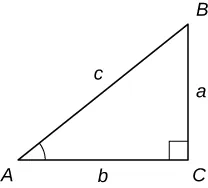129.

$a = 4 , c = 7 a = 4 , c = 7$

130.

$a = 21 , c = 29 a = 21 , c = 29$

131.

$a = 85.3 , b = 125.5 a = 85.3 , b = 125.5$

132.

$b = 40 , c = 41 b = 40 , c = 41$

133.

$a = 84 , b = 13 a = 84 , b = 13$

134.

$b = 28 , c = 35 b = 28 , c = 35$

For the following exercises, $PP$ is a point on the unit circle. a. Find the (exact) missing coordinate value of each point and b. find the values of the six trigonometric functions for the angle $θθ$ with a terminal side that passes through point $P.P.$ Rationalize denominators.

135.

$P ( 7 25 , y ) , y > 0 P ( 7 25 , y ) , y > 0$

136.

$P ( −15 17 , y ) , y < 0 P ( −15 17 , y ) , y < 0$

137.

$P ( x , 7 3 ) , x < 0 P ( x , 7 3 ) , x < 0$

138.

$P ( x , − 15 4 ) , x > 0 P ( x , − 15 4 ) , x > 0$

For the following exercises, simplify each expression by writing it in terms of sines and cosines, then simplify. The final answer does not have to be in terms of sine and cosine only.

139.

$tan 2 x + sin x csc x tan 2 x + sin x csc x$

140.

$sec x sin x cot x sec x sin x cot x$

141.

$tan 2 x sec 2 x tan 2 x sec 2 x$

142.

$sec x − cos x sec x − cos x$

143.

$( 1 + tan θ ) 2 − 2 tan θ ( 1 + tan θ ) 2 − 2 tan θ$

144.

$sin x ( csc x − sin x ) sin x ( csc x − sin x )$

145.

$cos t sin t + sin t 1 + cos t cos t sin t + sin t 1 + cos t$

146.

$1 + tan 2 α 1 + cot 2 α 1 + tan 2 α 1 + cot 2 α$

For the following exercises, verify that each equation is an identity.

147.

$tan θ cot θ csc θ = sin θ tan θ cot θ csc θ = sin θ$

148.

$sec 2 θ tan θ = sec θ csc θ sec 2 θ tan θ = sec θ csc θ$

149.

$sin t csc t + cos t sec t = 1 sin t csc t + cos t sec t = 1$

150.

$sin x cos x + 1 + cos x − 1 sin x = 0 sin x cos x + 1 + cos x − 1 sin x = 0$

151.

$cot γ + tan γ = sec γ csc γ cot γ + tan γ = sec γ csc γ$

152.

$sin 2 β + tan 2 β + cos 2 β = sec 2 β sin 2 β + tan 2 β + cos 2 β = sec 2 β$

153.

$1 1 − sin α + 1 1 + sin α = 2 sec 2 α 1 1 − sin α + 1 1 + sin α = 2 sec 2 α$

154.

$tan θ − cot θ sin θ cos θ = sec 2 θ − csc 2 θ tan θ − cot θ sin θ cos θ = sec 2 θ − csc 2 θ$

For the following exercises, solve the trigonometric equations on the interval $0≤θ<2π.0≤θ<2π.$

155.

$2 sin θ − 1 = 0 2 sin θ − 1 = 0$

156.

$1 + cos θ = 1 2 1 + cos θ = 1 2$

157.

$2 tan 2 θ = 2 2 tan 2 θ = 2$

158.

$4 sin 2 θ − 2 = 0 4 sin 2 θ − 2 = 0$

159.

$3 cot θ + 1 = 0 3 cot θ + 1 = 0$

160.

$3 sec θ − 2 3 = 0 3 sec θ − 2 3 = 0$

161.

$2 cos θ sin θ = sin θ 2 cos θ sin θ = sin θ$

162.

$csc 2 θ + 2 csc θ + 1 = 0 csc 2 θ + 2 csc θ + 1 = 0$

For the following exercises, each graph is of the form $y=AsinBxy=AsinBx$ or $y=AcosBx,y=AcosBx,$ where $B>0.B>0.$ Write the equation of the graph.

163.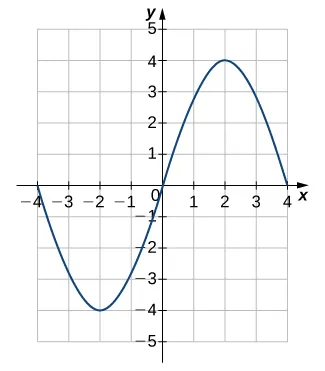164.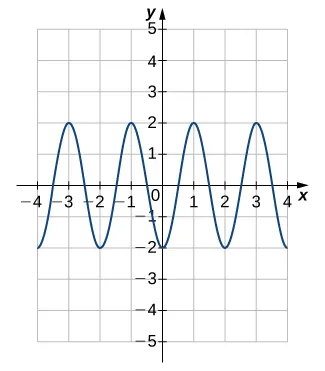165.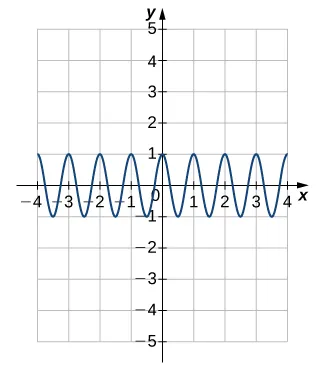166.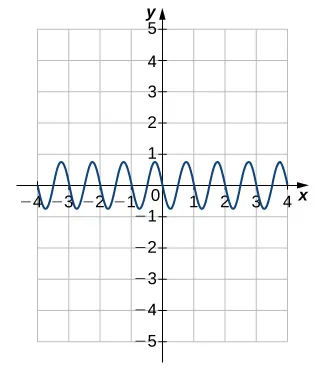For the following exercises, find a. the amplitude, b. the period, and c. the phase shift with direction for each function.

167.

$y = sin ( x − π 4 ) y = sin ( x − π 4 )$

168.

$y = 3 cos ( 2 x + 3 ) y = 3 cos ( 2 x + 3 )$

169.

$y = −1 2 sin ( 1 4 x ) y = −1 2 sin ( 1 4 x )$

170.

$y = 2 cos ( x − π 3 ) y = 2 cos ( x − π 3 )$

171.

$y = −3 sin ( π x + 2 ) y = −3 sin ( π x + 2 )$

172.

$y = 4 cos ( 2 x − π 2 ) y = 4 cos ( 2 x − π 2 )$

173.

[T] The diameter of a wheel rolling on the ground is 40 in. If the wheel rotates through an angle of $120°,120°,$ how many inches does it move? Approximate to the nearest whole inch.

174.

[T] Find the length of the arc intercepted by central angle $θθ$ in a circle of radius r. Round to the nearest hundredth.

a. $r=12.8r=12.8$ cm, $θ=5π6θ=5π6$ rad b. $r=4.378r=4.378$ cm, $θ=7π6θ=7π6$ rad c. $r=0.964r=0.964$ cm, $θ=50°θ=50°$ d. $r=8.55r=8.55$ cm, $θ=325°θ=325°$

175.

[T] As a point P moves around a circle, the measure of the angle changes. The measure of how fast the angle is changing is called angular speed, $ω,ω,$ and is given by $ω=θ/t,ω=θ/t,$ where $θθ$ is in radians and t is time. Find the angular speed for the given data. Round to the nearest thousandth.

a. $θ=7π4rad,t=10θ=7π4rad,t=10$ sec b. $θ=3π5rad,t=8θ=3π5rad,t=8$ sec c. $θ=2π9rad,t=1θ=2π9rad,t=1$ min d. $θ=23.76rad,t=14θ=23.76rad,t=14$ min

176.

[T] A total of 250,000 m2 of land is needed to build a nuclear power plant. Suppose it is decided that the area on which the power plant is to be built should be circular.

1. Find the radius of the circular land area.
2. If the land area is to form a $45°45°$ sector of a circle instead of a whole circle, find the length of the curved side.
177.

[T] The area of an isosceles triangle with equal sides of length x is

$1 2 x 2 sin θ , 1 2 x 2 sin θ ,$

where $θθ$ is the angle formed by the two sides. Find the area of an isosceles triangle with equal sides of length 8 in. and angle $θ=5π/12θ=5π/12$ rad.

178.

[T] A particle travels in a circular path at a constant angular speed $ω.ω.$ The angular speed is modeled by the function $ω=9|cos(πt−π/12)|.ω=9|cos(πt−π/12)|.$ Determine the angular speed at $t=9t=9$ sec.

179.

[T] An alternating current for outlets in a home has voltage given by the function

$V ( t ) = 150 cos 368 t , V ( t ) = 150 cos 368 t ,$

where V is the voltage in volts at time t in seconds.

1. Find the period of the function and interpret its meaning.
2. Determine the number of periods that occur when 1 sec has passed.
180.

[T] The number of hours of daylight in a northeast city is modeled by the function

$N ( t ) = 12 + 3 sin [ 2 π 365 ( t − 79 ) ] , N ( t ) = 12 + 3 sin [ 2 π 365 ( t − 79 ) ] ,$

where t is the number of days after January 1.

1. Find the amplitude and period.
2. Determine the number of hours of daylight on the longest day of the year.
3. Determine the number of hours of daylight on the shortest day of the year.
4. Determine the number of hours of daylight 90 days after January 1.
5. Sketch the graph of the function for one period starting on January 1.
181.

[T] Suppose that $T=50+10sin[π12(t−8)]T=50+10sin[π12(t−8)]$ is a mathematical model of the temperature (in degrees Fahrenheit) at t hours after midnight on a certain day of the week.

1. Determine the amplitude and period.
2. Find the temperature 7 hours after midnight.
3. At what time does $T=60°?T=60°?$
4. Sketch the graph of $TT$ over $0≤t≤24.0≤t≤24.$
182.

[T] The function $H(t)=8sin(π6t)H(t)=8sin(π6t)$ models the height H (in feet) of the tide t hours after midnight. Assume that $t=0t=0$ is midnight.

1. Find the amplitude and period.
2. Graph the function over one period.
3. What is the height of the tide at 4:30 a.m.?
Order a print copy

As an Amazon Associate we earn from qualifying purchases.